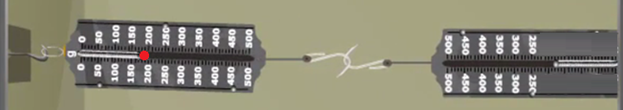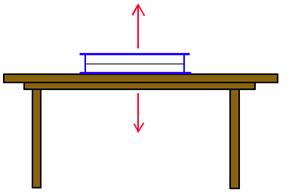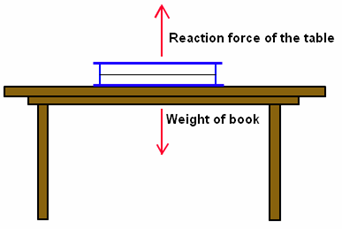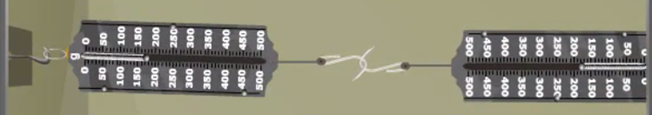# Third Law of Motion

## •    Newton’s third law of motion states that for every action there is an equal and opposite reaction. Examples of Newton’s third law of motion are walking, recoil of gun and rocket propulsion. •    Action and reaction forces always react on the different bodies. A man leaving a boat and a boy hitting a wall are also examples of Newton’s third law of motion.

To Access the full content, Please Purchase

• Q1

Name the scientist who stated the three laws of motion.

Marks:1
##### Answer:

Sir Isaac Newton stated the three laws of motion.

View Answer
• Q2

If the spring balance shows a reading of 200g, what will be the reading of another spring balance?Marks:1
##### Answer:

The reading of other spring balance will be 200 g.

View Answer
• Q3

Mark the action-reaction forces in the given diagram.Marks:1
##### Answer:View Answer
• Q4

Give two examples of Newton’s third law of motion.

Marks:1
##### Answer:

The two examples of Newton’s third law of motion are

(a) Recoil of a gun

(b) Motion of boat in water

View Answer
• Q5

Name the law applied in the picture shown below.Marks:1
##### Answer:

The law applied in the given picture is Newton’s third law of motion.

View Answer